Mathematics
Easy

Question

# Copy and complete the proof.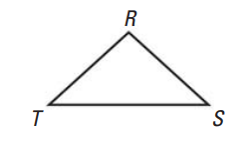GIVEN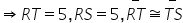PROVE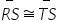What is reason 1 and reason 5? Statements Reasons 1.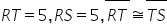1. ____ ? 2. RS = RT 2. Transitive Property of Equality 3. RT = TS 3. Definition of congruent segments 4. RS = TS 4. Transitive Property of Equality 5.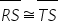5. _____?

## Reason 1 - Given, Reason 5 - Definition of midpointReason 1 - Definition of congruency, Reason 5 - GivenReason 1 - Given, Reason 5 - Definition of congruencyReason 1 - Definition of midpoint, Reason 5 - ConclusionHint:

## The correct answer is: Reason 1 - Given, Reason 5 - Definition of congruency

### We have to find the reason of 1st step and 5th step.Firstly , we are given a triangle RST , in which given is RT =5, RS = 5, (RT) ̅ ≅ (TS) ̅ .Now,Statement Reason1. RT =5, RS = 5, (RT) ̅ ≅ (TS) ̅ 1. Given2. RS = RT 2. Transitive property of Equality3. RT = TS 3. Definition of congruent segments4. RS = TS 4.Transitive Property of equality5. (RS) ̅ ≅ (TS) ̅ 5. Definition of CongruencyTherefore, the reason of step 1 is given and step 5 is definition of congruency.The correct answer is Reason 1 – Given , Reason 5 – Definition of Congruency.

In this question , we have to find the reason of step 1 and 5 , here step 1 is already given and step 5 is definition of congruency. Here we already proved that RS = TS so if two lines are equal then they are also congruent.

### Related Questions to study#### With Turito Foundation.#### Get an Expert Advice From Turito.